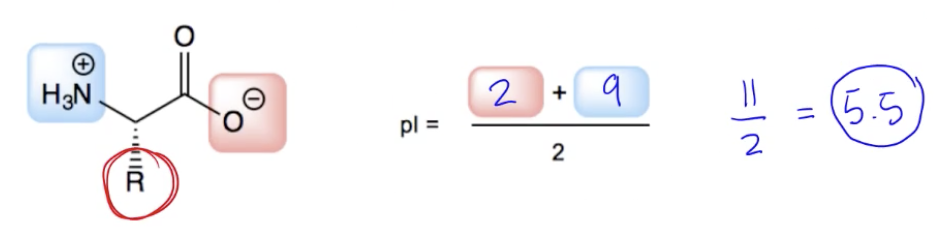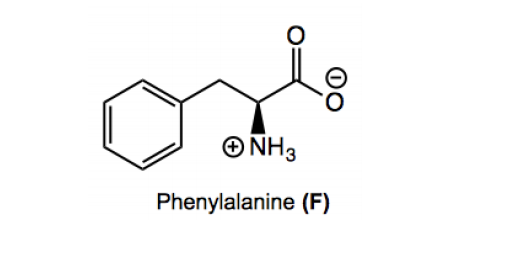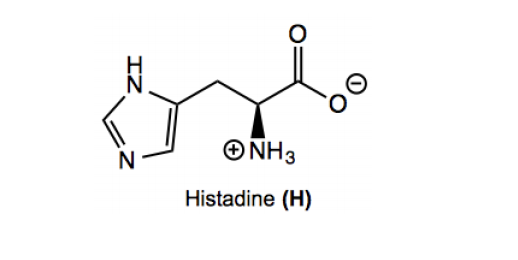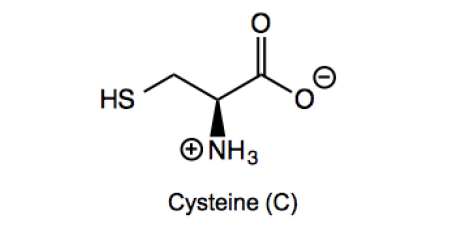Clutch Prep is now a part of Pearson
Ch. 26 - Amino Acids, Peptides, and ProteinsWorksheetSee all chapters

# Isoelectric Point

See all sections
Sections
Proteins and Amino Acids
L and D Amino Acids
Polar Amino Acids
Amino Acid Chart
Acid-Base Properties of Amino Acids
Isoelectric Point

In this section we will be doing small calculations in order to determine the isolectric point (pI) of various amino acids.

Concept #1: Definition of Isoelectric PointConcept #2: Isoelectric Point of Non-Charged Amino AcidsConcept #3: Isoelectric Point of Charged Amino AcidsConcept #4: Isoelectric Point of CysteinePractice: Calculate the isoelectric point of tyrosine (Y)

Practice: Calculate the isoelectric point of glutamic acid (E)